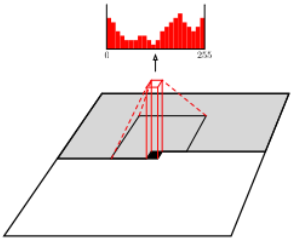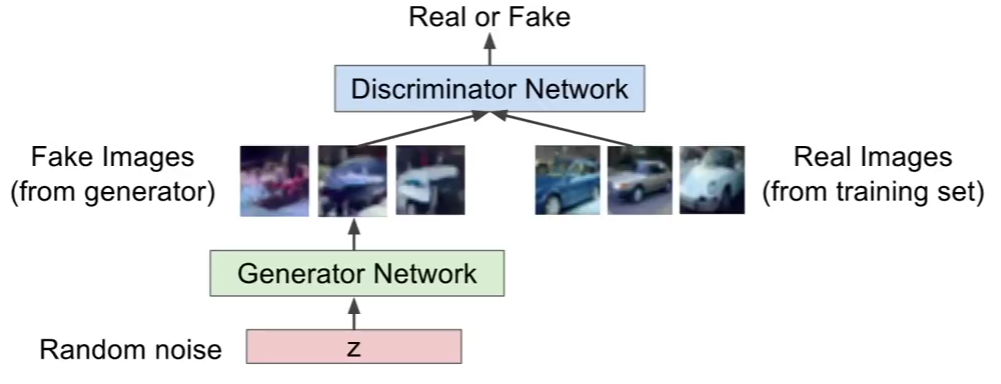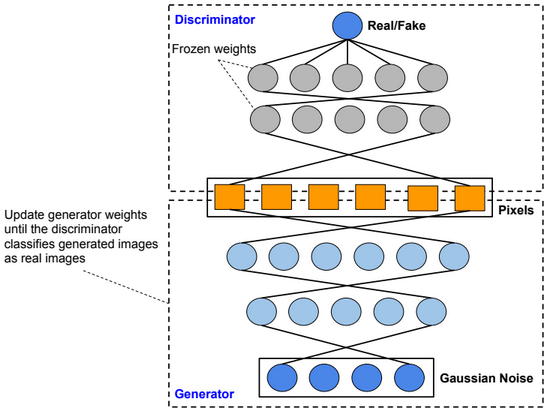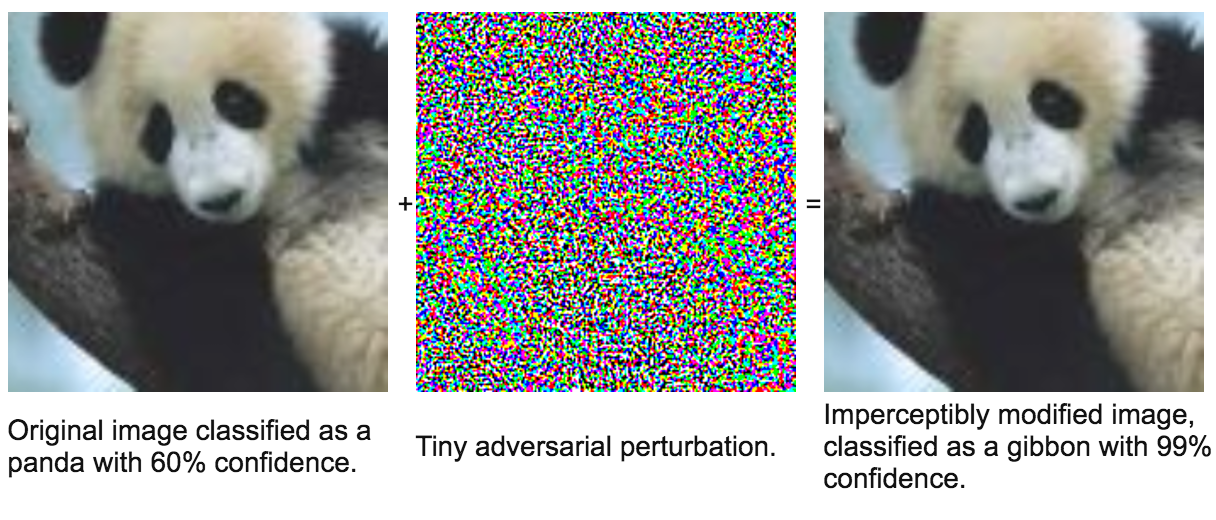# Deep Generative Models

Generative models is a class of unsupervised learning models.

Generative models estimate the density distribution of training data, and generate new samples from that distribution.

# PixelRNN

PixelRNN is a deep neural network that sequentially predicts/generates pixels in an image. The model estimates the density distribution of pixels by training a recurrent neural network.

$$p(image) = p(pixels) = \prod_{i=1}^{\#pixels} P(pixel_i|pixel_{i-1}, pixel_{i-2},…, pixel_0)$$

# PixelCNN

PixelRNN is accurate, but it is slow to train since RNN is hard to parallelize. PixelCNN is proposed to solve this problem. A mask is used to ensure convolution operation only uses previous pixels.# Generative Adversarial Networks (GAN)GANs use two neural networks to generate real looking images. The first network generates fake images, and the second network distinguishes between real and fake images.

GANs require an iterative training process in which we train consecutively the discriminator and the generator. When training the generator we freeze discriminator weights. and when training the discriminator we freeze generator weights.Deep Convolutional GANs

Deep convolutional GANs (DC-GANs) use convolutional layers instead of dense layers.

Conditional GANs

Conditional GANs are an extension of the GAN framework. More details will be provided sooner.

# Adversarial Examples

An adversarial example is an example which has been modified very slightly in a way that is intended to cause a machine learning classifier to misclassify it.Adversarial examples have the potential to be dangerous. For example, attackers could target autonomous vehicles by using stickers or paint to create an adversarial stop sign that the vehicle would interpret as a ‘yield’ or other sign.